# Find all indices of a given element in the sorted form of the given array

## Introduction

### Problem statement

We are given an array and a value K. Our task is to find all indices of K in the sorted form ascending) of the given array.

### Sample examples

Input1:  a[] = [ 3, 5, 7, 9, 7, 2, 7] , K = 7

Output1:  3 4 5   // all indices of 7

Explanation:

Sorted array: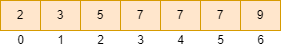Indices :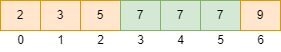Input2: a[] = [ 2, 3, 6, 4, 3, 4, 0] , K = 3

Output2:  2 3  // all indices of 3

Explanation:

Sorted array: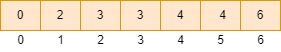Indices :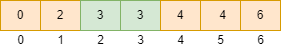## Naive Approach

The idea is straightforward: sort the given array and start traversing the array. Check for every element. If the element is K, print its index else move to the next element.

### Algorithm

• Sort the given array using the sort function sort(a, a+n), where n is the size of the array.
• Run a for loop for traversing the sorted array.
• If the current element is K, print its index.

### Implementation in C++

``````#include<bits/stdc++.h>
using namespace std;

void print_all_indices(int a[], int n, int K)
{
sort(a, a + n); // sorting the array

for (int i = 0; i < n; i++) // traversing the array
{
if (a[i] == K)
cout << i << " ";  // printing the index
}

cout << endl;
}

int main()
{
int a[] = {2, 3, 6, 4, 3, 4, 0};

int K = 3;

int n = sizeof(a) / sizeof(a);

print_all_indices(a, n, K);
}``````

Output:

``2 3``

#### Complexity Analysis

Time complexity: We are using the sort function in the above approach, so the time complexity is O(n*logn), where n is the number of elements in the given array.

Space complexity: O(1) requires constant extra space.

## Efficient Approach

We can improve the above solution by using the property of a sorted array, which states that if the array is sorted (ascending), the elements before the element with value K are smaller than K. The elements after the element with value K are greater than K.

Instead of sorting, we can use the above observation to find all indices of K in a sorted array.

If there are x elements smaller than K in the given array, the first index of K in the sorted array must be x (0-indexing).

For all indices of K, we count the number of elements having value K and print the indices x, x+1, x+2, x+3 ……. and so on till (x+ count of K - 1).

### Algorithm

• Traverse the given array
• Count the number of elements having a value less than K and store it in the count_smaller variable.
• Count the number of elements having a value equal to K and store it in the count_k variable.
• Run a for loop till count_k, print the count_smaller and keep incrementing the count_smaller variable.

For Example:

Input:  a[] = [ 3, 5, 7, 9, 7, 2, 7] , K = 7

Given array :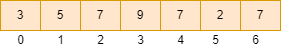Initially count_smaller = 0, count_k = 0.

Step 1: a = 3, count_smaller = 1, count_k = 0.

Step 2: a = 5, count_smaller = 2, count_k = 0.

Step 3: a = 7, count_smaller = 2, count_k = 1.

Step 4: a = 9, count_smaller = 2, count_k = 1.

Step 5: a = 7, count_smaller = 2, count_k = 2.

Step 6: a = 2, count_smaller = 3, count_k = 2.

Step 7: a = 7, count_smaller = 3, count_k = 3.

The indices are: count_smaller, count_smaller + 1, count_smaller + 2.

Indices = 3 4 5.

### Implementation in C++

``````#include<bits/stdc++.h>
using namespace std;

void print_all_indices(int a[], int n, int K)
{
int count_smaller = 0;
int count_k = 0;
for (int i = 0; i < n; i++)
{
if (a[i] < K)
count_smaller++;
if (a[i] == K)
count_k++;
}

for (int i = 0; i < count_k; i++)
{
cout << count_smaller << " ";
count_smaller++;
}
}

int main()
{
int a[] = {3, 5, 7, 9, 7, 2, 7};

int K = 7;

int n = sizeof(a) / sizeof(a);

print_all_indices(a, n, K);

return 0;
}``````

Output:

``3 4 5``

#### Complexity Analysis

Time complexity: We are traversing the given array, so the time complexity is O(n), where n is the number of elements in the given array.

Space complexity: O(1) requires constant extra space.

Q1. Which sorting method is the most appropriate for an array?

Ans: Insertion sort can be preferred when the array is almost sorted. Merge sort is preferred when the order of the input is unknown because it has a worst-case time complexity of n*logn and is also stable.

Q2. Where does quicksort come into play?

Ans: The sorting algorithm is used to find information, and because Quicksort is the fastest algorithm, it is widely used as a more efficient method of searching. It's used wherever a stable sort isn't required. Only traverses the arrayQuicksort has a good locality of reference when used for arrays, making it a cache-friendly algorithm.

Q3. Which sorting algorithm is best for large data?

Ans: Insertion sort is the fastest for large numbers of data sets. This is a rare occurrence in practical sorting. It's worth noting that randomised Quicksort reduces the likelihood of worst-case scenarios, which is the case for in-order data if the pivot point in Quicksort is set to the first element.

## Key Takeaways

This article discussed the naive approach and an efficient approach using the property of a sorted array for finding all indices of a given element in the sorted form of the given array with examples and its C++ code.

If you are a beginner, interested in coding and want to learn DSA, you can look for our guided path for DSA, which is free!